# Divide And Conquer

There's an old-school technique for rendering the classic fractal, the Mandelbrot set, called Divide and Conquer. This technique takes advantage of the fact that all of points in the Mandelbrot set are 'connected' - there are no islands of Mandelbrot set points which aren't connected to the main set; and there are no islands of non-Mandelbrot set enclosed by points within Mandelbrot set. Think of it as a single blob of water. Given this, you can speed up rendering of any subset of the Mandelbrot set by:

1. Divide the image into four rectangles.
2. For each of the four rectangles:
3. If the rectangle has a non-zero width and height:
4. Calculate all the points along the edges.
5. If all the points along the edges have the same calculated value, all points within the rectangle must be the same, too. No 'islands', remember. So, fill the rectangle with the value you calculated for the edges.
6. If the points along the edge are not the same, divide that rectangle into four rectangles and, for each sub-rectangle, go to step 3. (Keep in mind that since you've already calculated the points on the edges, you can reduce the size of the rectangle by 1 on each edge).

So, it's a recursive space-filling technique. It divides the work area into smaller and smaller rectangles until it finds something it can fill or it can no longer sub-divide. And in this way it gobbles-up large identically-valued sections of the Set very quickly, and so it spends most of its time working on details. In general, that's a performance gain. And, even better, this technique is far more fun to watch than just seeing pixels calculated one at a time, one row after another. It's kind of mezmerizing. Or, it was back in the day. These days, computers are fast enough that you can't see it in action.

Anyway, I thought it would be fun to put a variation of this technique to use on non-fractal images. So, I used color differences in the source image to determine where the edges of the rectangles go, with a selectable threshold to control color matching. I added a way to limit the minimum rectangle size, and taught it to optionally draw tastefully-colored borders around each rectangle.

And away we go!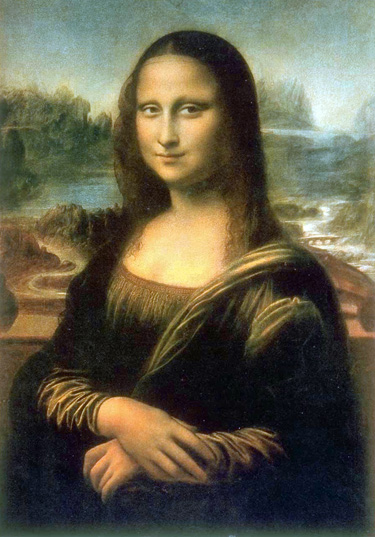Set the minimum rectangle dimension to 25 pixels, set the threshold to "100" and...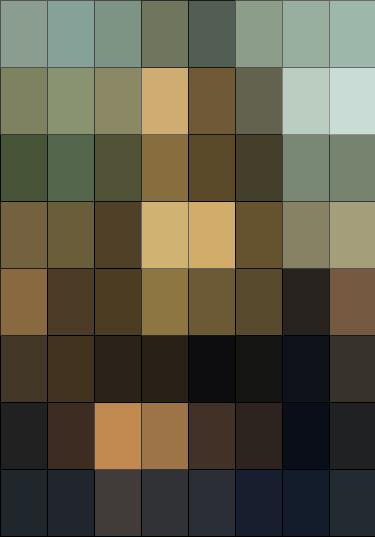Not amazing.

The rectangles are too big. So, reduce the min rect size to 10: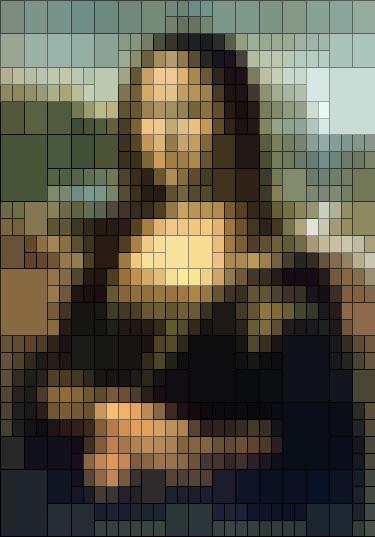Now you can start to see what's going on. Variety in rectangle sizes is what we're after - otherwise it's just pixelation. And this would be a most inefficient way to do pixelation...

Set min rect size to 4, and then to 2: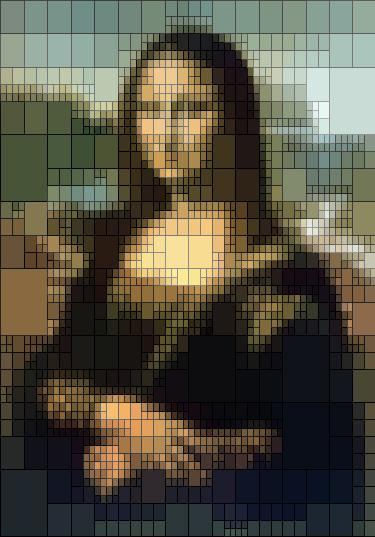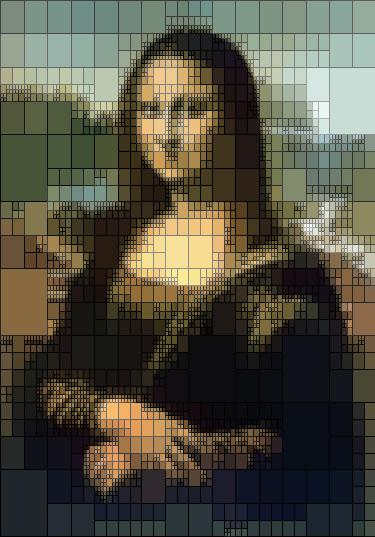There we go.

Now, that's fun.

But there's one thing I don't love about this: the rectangles follow a perfectly even grid, regardless of min rect size. Look at the four rectangles in the top-left of this one, then look at the previous images. They are the same in all of the images except for minsize=25 - and in that case, those four rects fit perfectly into its top-left rectangle. This is completely expected, since we're just dividing the image into 1/2 then 1/4 then 1/8 then 1/16, etc., and so we're going to keep hitting those same edges over and over.

What we need is to vary the sizes of the sub-rects, to get off of that power-of-two thing. To do that, I added something called 'jitter'. Instead of dividing the rectangle evenly into four equal sub-rects, jitter divides the rectangle by 2, 3 or 4 vertically, then 2, 3 or 4 horizontally. The divisor is chosen at random.

That gives: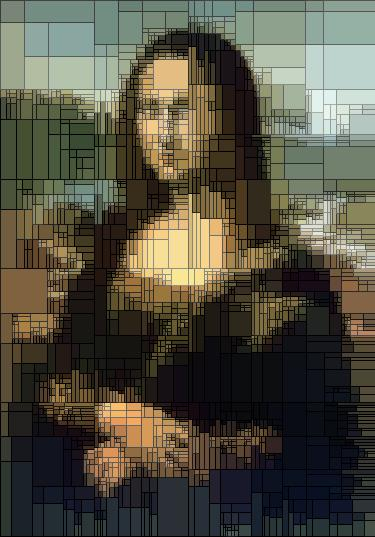And because the jitter is random, the output image will be different every time.

Other variations:

No outlines: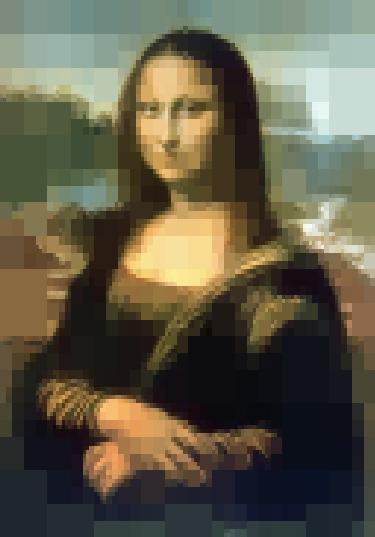Looks like hyper-aggressive JPEG compression.

Or, don't fill the rectangles, just draw the outlines: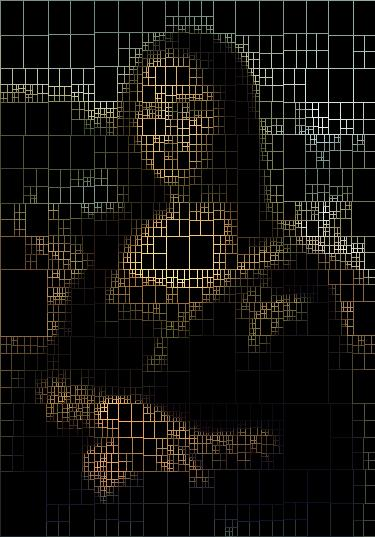I really like this effect. And this one shows another variation: instead of randomizing the sub-rect proportions, it just randomly nudges the edges of the sub-rects by a pixel or two. This maintains the even grid, but adds just enough noise to break the monotony if you look closely.

Or, render the same image twenty times with jitter on, and put the results into a GIF !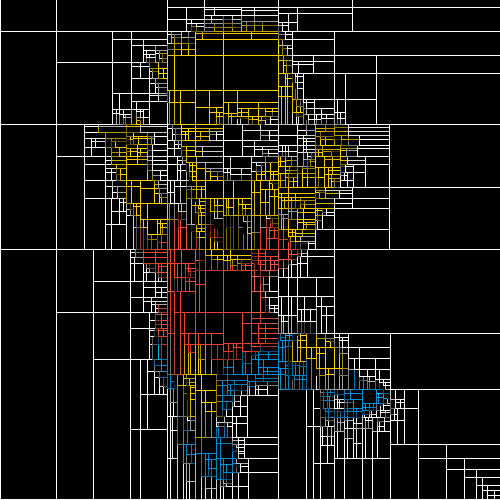Far out, man!

## 5 thoughts on “Divide And Conquer”

1.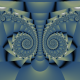cleek Post author

There’s a single black pixel in the bottom-right of the Bart image I use for that last one. And you can see the algorithm fighting to isolate it in different ways. Stupid computer.

2.Mike Mundy

You could make 20 versions of the Mona Lisa and print them up, 4′ by 6′ and have an exhibit at the Whitney, like Jeff Koons.

1.cleek Post author

do they have to be on one piece of paper? or can i tape a bunch of 8.5 x 11’s together?

3.ChrisR

That is fascinating and awesome. Very nice work!